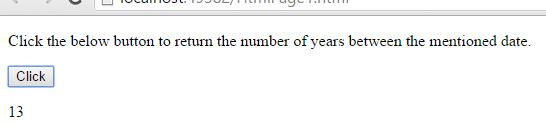# GetTime() Method in JavaScript

##### How to return the number of years since from the particular mentioned year in JavaScript?

By using `getTime()` method we can return the number of years since from the particular mentioned year.

Example: Calculating the number of years in between from 2002/October/13 to 2015/October/13.

```<p>Click the below button to return the number of years between the mentioned date.</p>
<input type="button" value="Click" onclick="myDate()" />
<p id="myId"></p>

<script>
function myDate() {
var min = 3600 * 60;
var hrs = min * 60;
var days = hrs * 24;
var years = days * 365;
var a = new Date();
var r = a.getTime();
var z = Math.round(r / years);
document.getElementById("myId").innerHTML = z;
}
</script>
```

In the above code snippet we have given `Id` as "`myId`"to the second `<p>` element in the HTML code. There is a function myDate() in the`<script>`block which is connected to the Onclick of the HTML button. The `document.getElementById(`"myId"`)`returns the element that has `Id`"id=myID" in the HTML page. We have added variables like `min`, `hrs`, `days`, `years` which are very useful to calculate the time period. In the `<script>` block var `hrs` indicates that there are 60 minutes per an hour, var `dyas` indicates 24 hours per a day, var `year` indicates 365 days per an year.

There is var `a` which returns the local time, date, day, timezon, var `r` returns the number of milliseconds since form the date 2002/oct/13 to present date, var `z` caluclate and return the number of years are there in between the date 2002/oct/13. Onclick of the button "Click" fires the function mydate() in the `<script>` block at the same time `getTime()` returns the number of milliseconds from that `var z = Math.round(r / years)` returns the number of years as output.

OUTPUTViews: 2566 | Post Order: 107